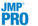Basic Analysis > Oneway Analysis > Nonparametric Tests > The Wilcoxon, Median, Van der Waerden, and Friedman Rank Test Reports
Publication date: 07/30/2020

## The Wilcoxon, Median, Van der Waerden, and Friedman Rank Test Reports

For each test, the report shows the descriptive statistics followed by the test results. Test results appear in the 1-Way Test, ChiSquare Approximation report and, if the X variable has exactly two levels, a 2-Sample Test, Normal Approximation report also appears. The descriptive statistics are the following:

Level

The levels of X.

Count

The frequencies of each level.

Score Sum

The sum of the rank score for each level.

Expected Score

The expected score under the null hypothesis that there is no difference among class levels.

Score Mean

The mean rank score for each level.

(Mean-Mean0)/Std0

The standardized score. Mean0 is the mean score expected under the null hypothesis. Std0 is the standard deviation of the score sum expected under the null hypothesis. The null hypothesis is that the group means or medians are in the same location across groups.

### 2-Sample Test, Normal Approximation

When you have exactly two levels of X, a 2-Sample Test, Normal Approximation report appears. This report gives the following:

S

Gives the sum of the rank scores for the level with the smaller number of observations.

Z

Gives the test statistic for the normal approximation test. See Two-Sample Normal Approximations.

Prob>|Z|

Gives the p-value, based on a standard normal distribution, for the normal approximation test.

### 1-Way Test, ChiSquare Approximation

This report gives results for a chi-square test for location. See Conover (1999).

ChiSquare

Gives the values of the chi-square test statistic. See One-Way ChiSquare Approximations.

DF

Gives the degrees of freedom for the test.

Prob>ChiSq

Gives the p-value for the test. The p-value is based on a ChiSquare distribution with degrees of freedom equal to the number of levels of X minus 1.

###2-Sample, Exact Test

If your data are sparse, skewed, or heavily tied, exact tests might be more suitable than approximations based on asymptotic behavior. When you have exactly two levels of X, JMP Pro computes test statistics for exact tests. Select Nonparametric > Exact Test and select the test of your choice. A 2-Sample: Exact Test report appears. This report gives the following:

S

Gives the sum of the rank scores for the observations in the smaller group. If the two levels of X have the same numbers of observations, then the value of S corresponds to the last level of X in the value ordering.

Prob S

Gives a one-sided p-value for the test.

Prob ≥ |S-Mean|

Gives a two-sided p-value for the test.